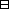Proof Machine

A talk at University of Melbourne 7 March 2014

 Assume the ifs To show: the thens

Proof type II:    To show: If   A   then   B  .

The next lines are

• Assume   A  .
• To show:   B  .

Let $f:S\to T$ be a function. Show that $f$ is invertible $⟺\text{if and only if}\forall \epsilon >0\exists \delta >0\ni x\in ℝ&|x|<\delta ⇒|{e}^{x}-1|<\epsilon$ $f$ is a bijection.Proof. To show: (a) If $f$ is invertible then $f$ is a bijection. $\phantom{\text{To show:}}$ (b) If $f$ is a bijection then $f$ is invertible. $\phantom{T}$ (a) Assume $f$ is invertible. $\phantom{\text{(a)}}$ To show: $f$ is a bijection. $\phantom{T}$ $\phantom{\text{(a)}}$ We know: There exists $g:T\to S$ such that $g∘f=idS and f∘g=idT.$ $\phantom{T}$ $\phantom{\text{(a)}}$ To show: (aa) $f$ is injective. $\phantom{\text{(a)}}$ $\phantom{\text{To show:}}$ (ab) $f$ is surjective. $\phantom{T}$ $\phantom{\text{(a)}}$ (aa) To show: If ${s}_{1},{s}_{2}\in S$ and $f\left({s}_{1}\right)=f\left({s}_{2}\right)$ then ${s}_{1}={s}_{2}$. $\phantom{T}$ $\phantom{\text{(a)}}$ $\phantom{\text{(aa)}}$ Assume ${s}_{1},{s}_{2}\in S$ and $f\left({s}_{1}\right)=f\left({s}_{2}\right)$. $\phantom{\text{(a)}}$ $\phantom{\text{(aa)}}$ To show: ${s}_{1}={s}_{2}$.               (proof type I) $\phantom{T}$ $s1= idS(s1)= g (f(s1)) = g (f(s2)) = idS(s2)= s2.$ $\phantom{\text{(a)}}$ So $f$ is injective. $\phantom{T}$ $\phantom{\text{(a)}}$ (ab) To show: $f$ is surjective. $\phantom{T}$ $\phantom{\text{(a)}}$ $\phantom{\text{(ab)}}$ To show: If $t\in T$ then there exists $s\in S$ such that $f\left(s\right)=t$. $\phantom{T}$ $\phantom{\text{(a)}}$ $\phantom{\text{(ab)}}$ Assume $t\in T$. $\phantom{\text{(a)}}$ $\phantom{\text{(ab)}}$ To show: There exists $s\in S$ such that $f\left(s\right)=t$.               (proof type III) $\phantom{T}$ $\phantom{\text{(a)}}$ $\phantom{\text{(ab)}}$ Define $s=g\left(t\right)$. $\phantom{\text{(a)}}$ $\phantom{\text{(ab)}}$ To show: $f\left(s\right)=t$. $\phantom{T}$ $f(s) =f(g(t)) =idT(t) =t.$ $\phantom{T}$ $\phantom{\text{(a)}}$ So $f$ is surjective. $\phantom{T}$ So $f$ is bijective. $\phantom{T}$ $\phantom{T}$ (b) To sh.... . . . . $\square$

Proof type I:   LHS = RHS.

The next lines are

LHS = ⋯ = ⋯ = ⋯ = ⋯ = $\text{blah}$   .
RHS = ⋯ = ⋯ = ⋯ = ⋯ = $\text{blah}\text{EXACTLY THE SAME blah}$   .

Prove that ${e}^{x+y}={e}^{x}{e}^{y}$.Proof. $ex+y = 1 + (x+y) + (x+y)2 2 + (x+y)3 3! +⋯ T = 1+ x+y+ x22 + 2xy2 + y22 + x3 3⋅2 + 3x2y 3⋅2 + 3xy2 3⋅2 + y3 3⋅2 + ⋮ T = ex+ exy+ exy2 2+ exy3 3⋅2+ ⋯ = ex( 1+y+ y2 2+ y3 3!+ ⋯) = ex ey.$ $\square$

Proof type III:   There exists   C   such that   D  .

The next lines are

• Define   C = xxx  .
• To show:   D  .

Prove that ${e}^{x}$ is continuous at 0.Proof. To show: $\underset{x\to 0}{\mathrm{lim}}{e}^{x}={e}^{0}$. $\phantom{T}$ To show: $\underset{x\to 0}{\mathrm{lim}}{e}^{x}=1$. $\phantom{T}$ To show: If $\epsilon \in {ℝ}_{>0}$ then there exists $\delta \in {ℝ}_{>0}$ such that if $|x-0|<\delta$ then $|{e}^{x}-1|<\epsilon$. $\phantom{T}$ Assume $\epsilon \in {ℝ}_{>0}$. To show: There exists $\delta \in {ℝ}_{>0}$ such that if $|x-0|<\delta$ then $|{e}^{x}-1|<\epsilon$. $\phantom{T}$ Define $\delta =\mathrm{?????}\frac{1}{2}\mathrm{min}\left(\frac{\epsilon }{2},\frac{1}{2}\right)$. To show: If $|x-0|<\delta$ then $|{e}^{x}-1|<\epsilon$. $\phantom{T}$ Assume $|x|<\delta$. To show: $|{e}^{x}-1|<\epsilon$. $\phantom{T}$ $|ex-1| = | (1+x+ x22! + x33! +⋯) -1| = | x+ x22! + x33! +⋯ | = | x| ⋅ | 1+ x2! + x23! +⋯ | ≤ | x| ⋅ | 1+ |x| 2! + |x|23! +⋯ | ≤ |x| ⋅ | 1+ |x| + |x|2 +⋯ | ≤ |x| ⋅ ( 1+ |x| + |x|2 +⋯ ) < δ ⋅ ( 1+ δ + δ 2 +⋯ ) ≤ δ ⋅ ( 1+ 12 + (12) 2 +⋯ ) ≤ δ ⋅ 2 ≤ ε2 ⋅ 2 = ε.$ $\square$

Special kinds of proofs

1. Proofs of uniqueness    (${x}_{1}={x}_{2}$)
$\phantom{T}$
$\phantom{T}$
3. Proofs of presentations (generators and relations)
$\phantom{T}$
4. Proofs by induction (the definition of ${ℤ}_{>0}$!!!)
$\phantom{T}$
5. Proof by intimidation (the most common and least rigourous)
$\phantom{T}$

Definitions

1. Format:
1. A noun is an   object   such that condition.

2. An adjective noun is a       such that condition.

3. A   condition   or   statement   can only be in the form
1.
If   A   then   B
or
There exists   C   such that   condition  .

2. Definitions can change!
$\phantom{T}$
1. "What's the definition of a Schur function?!?!!"
$\phantom{T}$
3. The challenge of taking on a definition.
$\phantom{T}$

Extra stuff

1. How did you come to formalise Proof Machine?
$\phantom{T}$
2. My notes page: Google "Arun Ram Notes"

top

The medical doctor

Before you try Lindelöf we need to an $\text{ERA}\text{Excellence in Research for Australia}$ assessment on your $\text{ARC}\text{Australian Research Council}$ results to check that there is no underlying $\text{NSF}\text{National Science Foundation}$ in the $\text{IMU}\text{International Mathematical Union}$. Believe me, ending up Lipschitz is no fun for anyone.